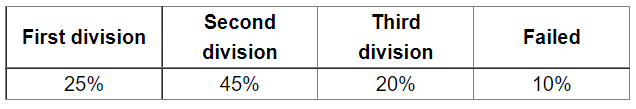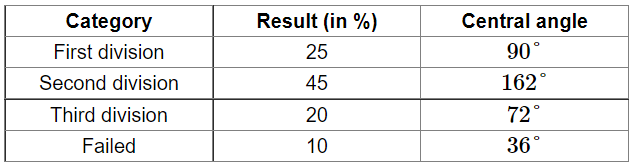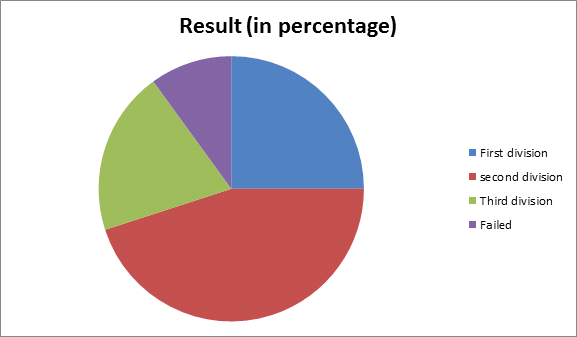# Given below is the result of an annual examination of a class, showing the percentage of students in each category.

Question:

Given below is the result of an annual examination of a class, showing the percentage of students in each category.Represent the above data by a pie chart.

Solution:

Total percentage $=100$

Central angle of each category $=\left(\frac{\text { value (in \%) of each category }}{100} \times 360\right)^{\circ}$

Calculation of central anglesConstruction of pie chart

Steps of construction:

1. Draw a circle of any convenient radius.

2. Draw a horizontal radius of the circle.

3. Starting from the horizontal radius, draw sectors whose central angles are $90^{\circ}, 162^{\circ}, 72^{\circ}$ and $36^{\circ}$.

4. Shade the sectors so obtained differently and label each one of them.

Thus, we obtain the required pie chart as shown in the figure below.